• 最短路径算法是图论中的常见问题，在实际中有着较为广泛的应用，比如查找从一个地方到另一个地方的最快方式。问题可以概括为，对于某个输入顶点s，给出s到所有其它顶点的最短路径。水平有限，暂时先对这个问题的求解...
  最短路径算法是图论中的常见问题，在实际中有着较为广泛的应用，比如查找从一个地方到另一个地方的最快方式。问题可以概括为，对于某个输入顶点s，给出s到所有其它顶点的最短路径。水平有限，暂时先对这个问题的求解做简单记录。
无权图是有权最短路径的特例，即边的权重均是1。算法类似于BFS（宽度优先搜索），在实现时需要一个宽度优先搜索的队列。全局变量Distance用来保存所有点到输入顶点的距离。以邻接表表示图，无权图最短路径算法：

//无权图的最短路径算法
static void UnweightedShortestPath(Graph g, int start){
int v,w;
Q.offer(start);
g.getVertics().get(start).setVivited(true);  //输入顶点被标记为已访问
for(int i=0;i<g.getVertexCount();i++)
Distance[i] = -1;   //初始化Distance，都为-1
Distance[start] = 0;
while(!Q.isEmpty()){
v = Q.remove();    //v是队首的整数，记录了图中顶点的序号
g.getVertics().get(w).setVivited(true);  //图的对应部分标记为已访问,每个顶点只能被访问一次
if(Distance[w] == -1){
Distance[w] = Distance[v]+1;
Path[w] = v;
Q.offer(w);
}
}//while
}//while
}  Dijkstra算法是解决最短路径问题的常见算法，过程需要使用优先队列来代替无权图最短路径算法。源点到某个顶点的距离为从源点到该顶点的路径上的所有边权值之和，当新计算得到的距离小于原有的距离时，更新距离。

//Dijkstra算法
static void Dijkstra(Graph g, int start){
MinHeap PQ = new MinHeap(10, 0);     //最小堆实现优先队列
int v,w;
PQ.Insert(new NodeWithProority(0,0));//NodeWithprority是一个只包括顶点的序号和权值（到给定起始点的距离）的类
g.getVertics().get(start).setVivited(true);
for( int i=0;i<g.getVertexCount();i++ )
Distance[i]=-1;
Distance[start] = 0;
while(!PQ.isEmpty()){
v = PQ.DeleteMin().node;
g.getVertics().get(w).setVivited(true);  //图的对应部分标记为已访问,每个顶点只能被访问一次
int d = Distance[v]+g.Weight(v, w);      //计算新的距离
if(Distance[w] == -1){
Distance[w] = d;
PQ.Insert(new NodeWithProority(w, d));
Path[w] = v;
}//if
if(Distance[w] > d){                    //如果新的距离比原有的小，需要更新距离
Distance[w] = d;
for(int i=0;i<PQ.count;i++){
if(PQ.array[i].node == w){
PQ.array[i].priority = d;
PQ.Parent(0);
}
}
Path[w] = v;
}//if
}//while
for(int j=0;j<g.getVertexCount();j++)  //将访问标记初始化
g.getVertics().get(j).setVivited(false);
}//while
}


展开全文数据结构复习 优先队列和堆 dijkstra
• BFS 基于队列实现，DFS 基于栈实现，是最基本的图算法，但是它们的扩展有很多实际用处。下面给出这两种搜索的实现： def bfs(graph, v): queue = [v] visited = set() visited.add(v) res = [] while queue !...
BFS 基于队列实现，DFS 基于栈实现，是最基本的图算法，但是它们的扩展有很多实际用处。下面给出这两种图搜索的实现：
def bfs(graph, v):
queue = [v]
visited = set()
res = []
while queue != []:
temp = queue.pop(0)
res.append(temp)
for neighbor in graph[temp]:
if neighbor not in visited:
queue.append(neighbor)
return res

def dfs(graph, v):
stack = [v]
visited = set()
res = []
while stack != []:
temp = stack.pop()
res.append(temp)
for neighbor in graph[temp]:
if neighbor not in visited:
stack.append(neighbor)
return res

if __name__ == '__main__':
g = {'A': ['B', 'C'],
'B': ['A', 'C', 'D'],
'C': ['A', 'D', 'E'],
'D': ['B', 'C', 'E', 'F'],
'E': ['C', 'D'],
'F': ['D']}
print(bfs(g, 'F'))
print(dfs(g, 'F'))

这里使用了 Python 的 set 容器记录已经访问过的顶点。下面简单扩展一下 BFS，实现一个寻找无权图中任意两点最短路径的算法：
def shortestPath(graph, v, target):
queue = [v]
visited = set()
res = {}
while queue != []:
parent = queue.pop(0)
for neighbor in graph[parent]:
if neighbor not in visited:
queue.append(neighbor)
res[neighbor] = parent
key = target
path = [target]
while key is not v:
path.append(res[key])
key = res[key]
return path[::-1]

if __name__ == '__main__':
g = {'A': ['B', 'C'],
'B': ['A', 'C', 'D'],
'C': ['A', 'D', 'E'],
'D': ['B', 'C', 'E', 'F'],
'E': ['C', 'D'],
'F': ['D']}
print(shortestPath(g, 'F', 'C'))

对于一个无权图来说，实际上从一点 BFS 到其他点的搜索路径就是这两点间的最短路径，只要使用一个映射（这里是一个字典）记录过程中每个顶点的 parent，最后逆向打印出来就可以了。
展开全文•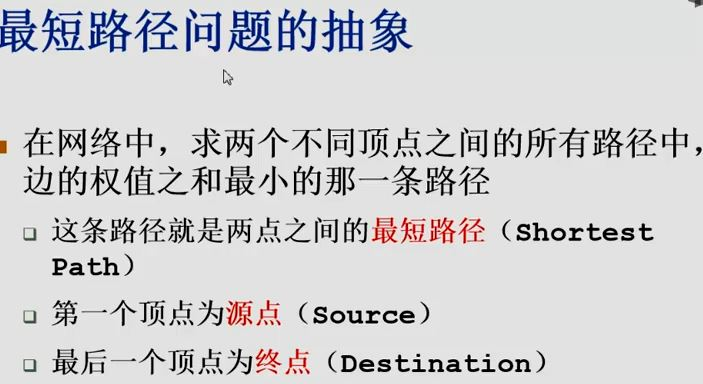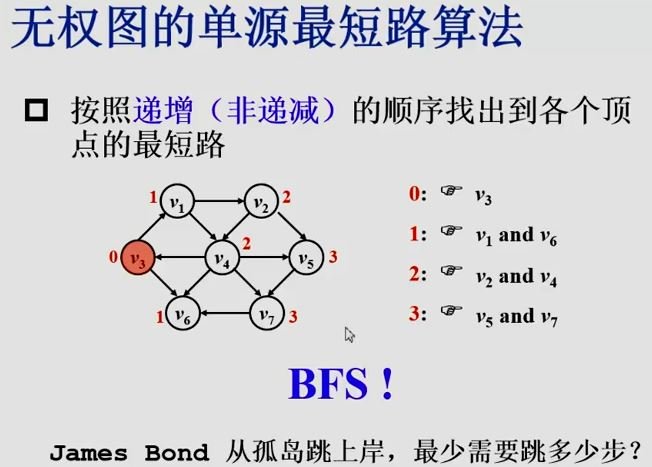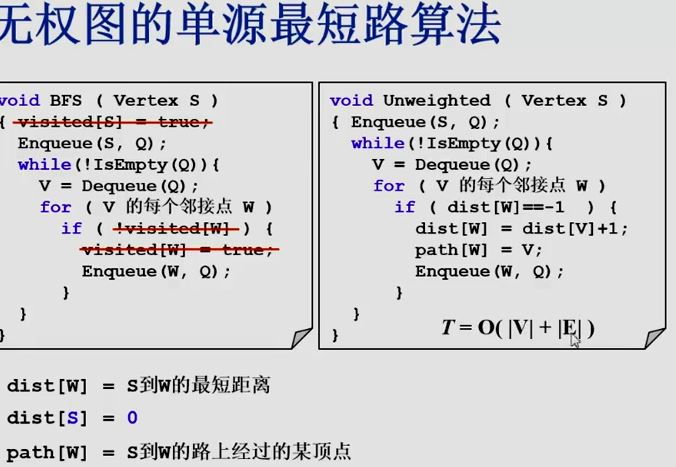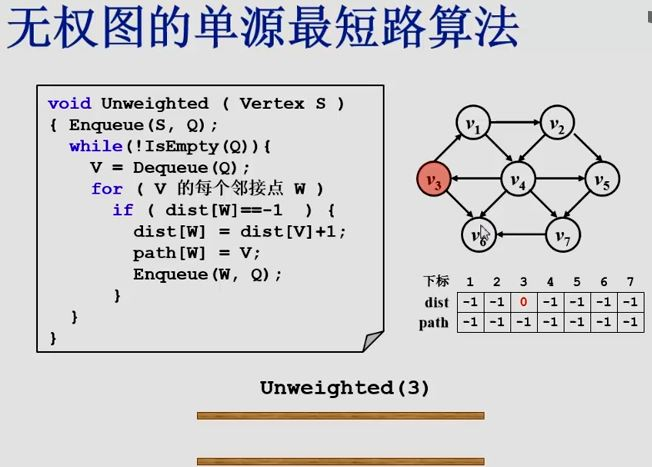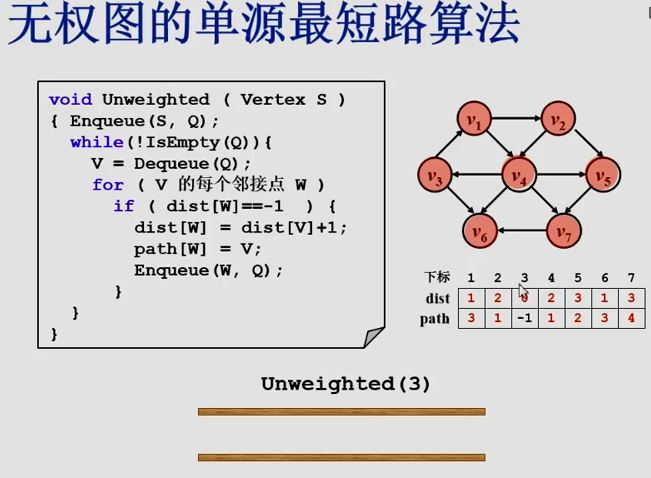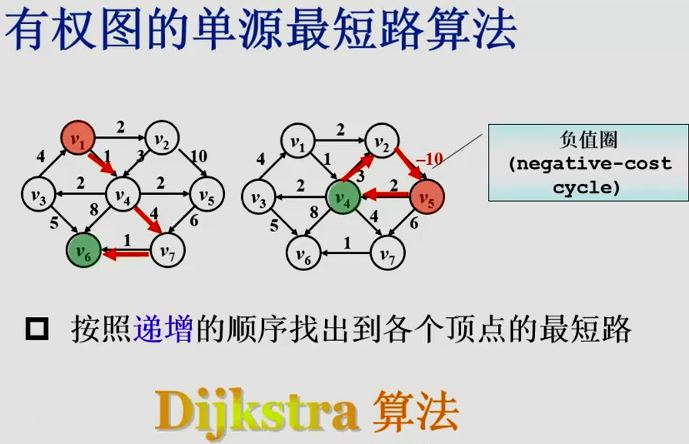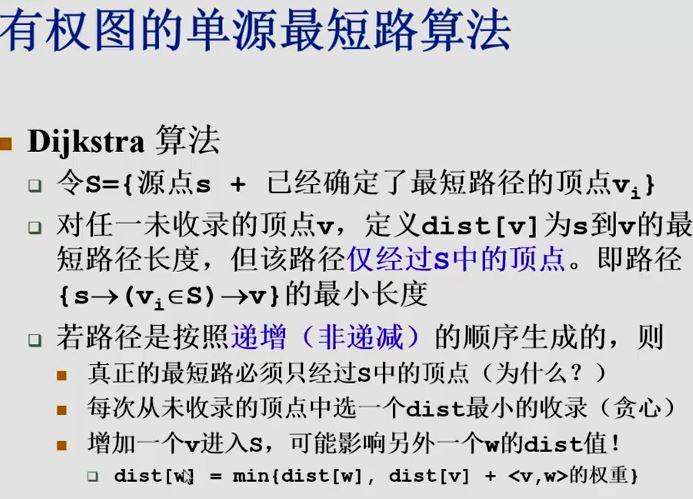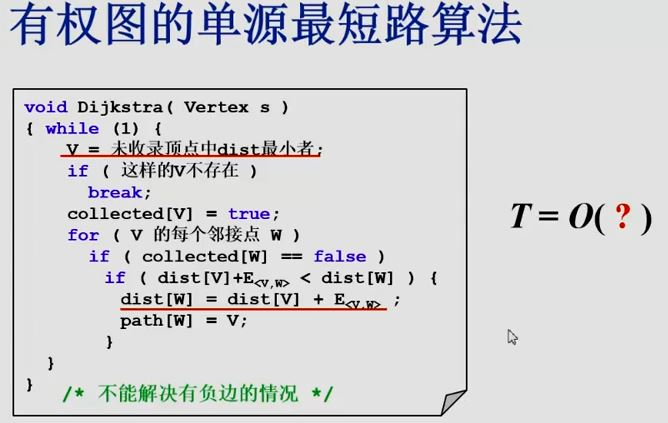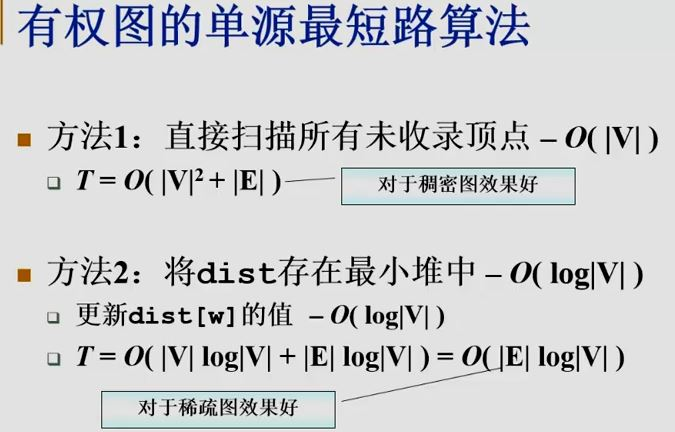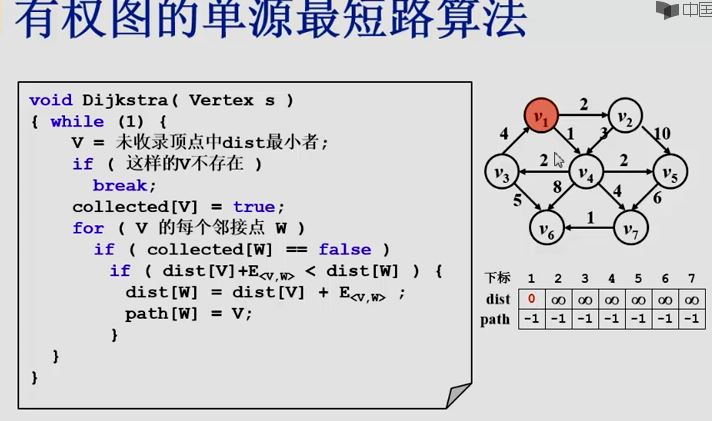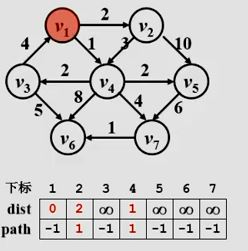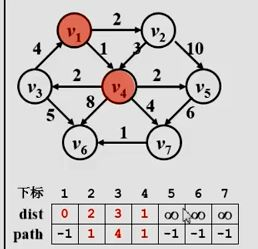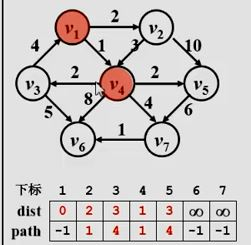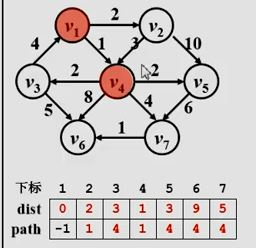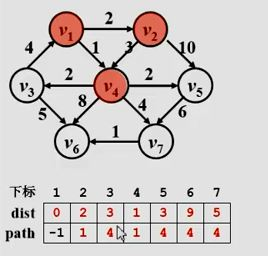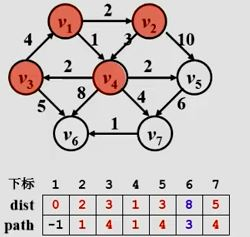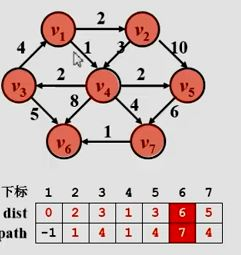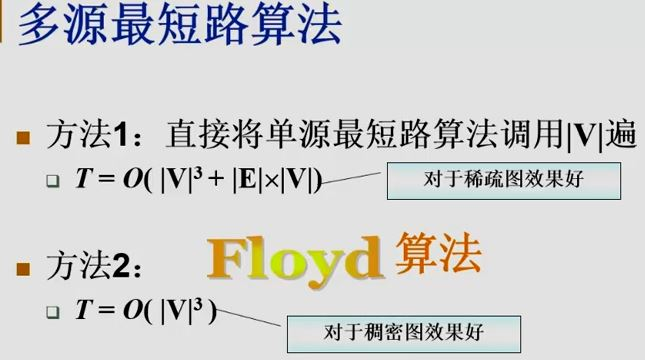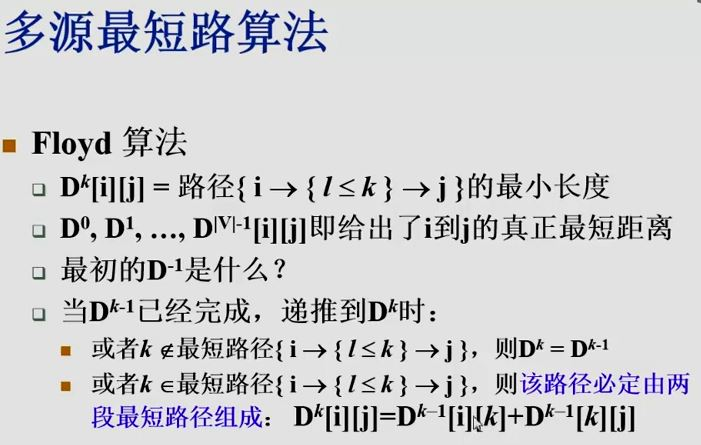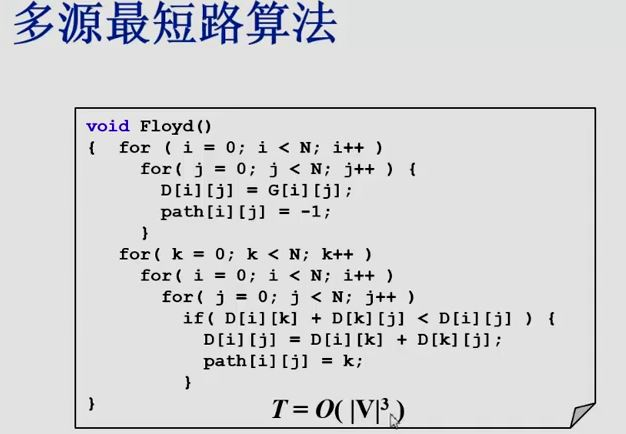展开全文• 其中又分为无权最短路径，单源最短路径，具有负边的最短路径以及无圈等；而这次将介绍常见的两个——无权最短路径以及单源最短路径。接下来就开始我们的讲解吧~~ 首先还是惯例来一个：Github传送门~~原理 最短...
前言

今天将给大家介绍的是图论算法中的另外一个基础部分——最短路径算法；其中又分为无权最短路径，单源最短路径，具有负边的最短路径以及无圈图等；而这次将介绍常见的两个——无权最短路径以及单源最短路径。接下来就开始我们的讲解吧~~

首先还是惯例来一个：
Github传送门~~

原理
最短路径算法，顾名思义是一种用来找出从某地到另外某个地方所经过的路径长度最短的算法，如图一所示。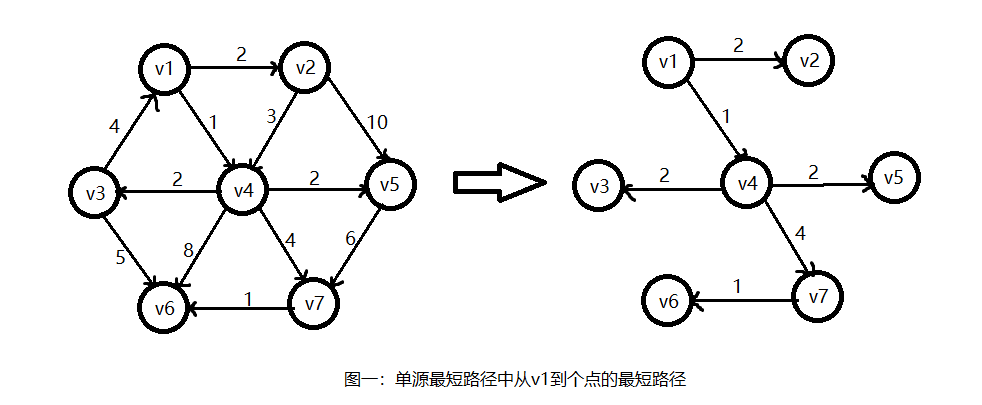上图中所展示的正是Dijkstra算法查找后的结果，我们可以清楚的了解从v1点开始，到其他点的最短路径该如何选择，以及其所经过的没条边的权重；接下来我将具体介绍其实现方式：

无权最短路径：

在无权图中因为边上不存在权重，因此我们可以把每条边的权重都当做1。当我们从某个顶点开始检索的时候，我们将该节点
压入一个队列中或者是栈中（当然你也可以通过for循环来检索，不过时间复杂度会上升到O(n * n) ），然后将该节点标位已知节点，接下来检索所有与该节点相连接的节点，更新他们的路径与距离，并标位已知，接下来重复刚才的步骤，只不过
我们只对未更新过的节点（即距离无穷的节点）进行操作，直到所有节点都已知。

以下是伪代码：

int Unweighted(vertex start) {
queue Q;
vertex v, w;

enqueue(start);
// 遍历所有的节点
while(all vertex is retrieved) {
v = dequeue;
v is known;

// 遍历相邻节点
for each w adjacent to v
// 更新路径
if Dist to w is infinity {
Dist to w = Dist to v + 1;
Path to w is v;
enqueue(w);
}
}
}

伪代码中有几点需要说明：

1.遍历所有节点我们只需要保证当队列是空时即可，这和拓扑排序很相似，大家可以想一想；

2.更新路径时我们只需要保证路径未被更新过即可进行更新，因为只要我们
更新过得路径即为最短路径，关于这一点大家也可以想一想；

2.Dijkstra算法：

在赋权图中情况看似变得很复杂了，但其实我们用和无权图中相似的思想，也可以解决它，这就是我接下来介绍的Dijkstra算法；同上面类似，我们也从某个顶点开始检索将其标位已知，并更新其链接的顶点的路径信息，不同之处是，我们
选择未知节点中路径距离最短的作为下一次检索的起始顶点，然后一直进行检索直到所有顶点都已知；

以下是伪代码：

int Dijkstra(vertex start) {
vertex v, w;

// 遍历所有的节点
while(true) {
v = smallest unknown distance vertex;
if v = novertex
break;

v is known;
// 遍历相邻节点
for each w adjacent to v
// 更新路径
if(w is unknown && Dist to v + v->w < Dist to w) {
Dist to w = Dist ti v + v->w;
Path to w is v;
}
}
}

当然上诉代码中也有几点需要注意的地方：

1.查找最短距离未知节点的方法会直接影响到我们的时间复杂度，最好的方法是使用优先队列来完成；当然使用不同优先队列也会有差距，其中我们按照时间复杂度来排序的话：斐波拉契堆(O(E + V * logV)) < 配对堆(O(E * logV)) < 二叉堆(O(E * logV + V * logV))；

2.
查找最短距离未知节，并以此节点开始检索也是算法中最重要的东西，他保证了我们每次更新的起始节点v的距离一定是最短的，从而保证了算法的正确性；

C++实现：

最后给出的是整个实现的代码，按照惯例先是.h文件：

#ifndef ALGRAPH_H
#define ALGRAPH_H

#include <iostream>
#include <iomanip>
#include <queue>
using namespace std;

// 重定义边节点，便于操作
typedef struct ArcNode *Position;

/* 边节点
* 储存元素:
* Weight:该有向边的权重
* Next:该有向边头节点的其他边节点
*/
struct ArcNode {
int Weight;
Position Next;
};

/* 顶点节点
* 储存元素:
* Name:该节点的姓名；
* firstArc:该顶点链接的第一个有向边；
*/
struct VexNode {
int Name;
Position firstArc;
};

/* 表节点
* 储存元素:
* Known:该节点检测状态；
* Dist:该节点到目标节点的最小距离；
* Path:最小距离时其链接的上一个节点；
*/
struct TNode {
bool Known;
int Dist;
int Path;
};

/* ALGraph类
* 接口:
* Creat:创建功能，在选定节点之间创建有向边；
* MakeEmpty:置空功能，将所有有向边删除，初始化各个顶点；
* Unweighted:排序功能，找出选定顶点对于其他顶点的最短无权路径；
* Display:展示功能，展示该图的路径信息；
* Dijkstra:Dijkstra算法，用于计算赋权图最短路径
* WeightNegative:排序功能，用于计算具有负边值的图的最短路径
*/
class ALGraph
{
public:
// 构造函数
ALGraph(int = 10);
// 析构函数
~ALGraph();

// 接口函数
// 基础函数
void Creat();
void MakeEmpty();
void Display();

// 最短路径函数
void Unweighted(int);
void Dijkstra(int);
void WeightNegative(int);

private:
// 辅助函数
void InitTable();

// 数据成员
int VexNum; // 储存顶点数
int ArcNum; // 储存边数
TNode *Table; // 储存距离表
};

#endif // !ALGRAPH_H 

然后是.cpp文件，不过在这个文件中还有计算带负边的有向图的最短路径算法，有兴趣的朋友可以自己看下：

#include "stdafx.h"
#include "ALGraph.h"

/* 构造函数:初始化对象
* 返回值:无
* 参数:vnum:图中需要的顶点数
*/
ALGraph::ALGraph(int vnum)
: VexNum(vnum), ArcNum(0){
// 申请邻接表
AdjList = new VexNode[VexNum + 1];
// 申请距离表
Table = new TNode[VexNum + 1];

// 判断是否申请成功
if (AdjList == NULL || Table == NULL)
cout << "邻接表申请失败!" << endl;

else {
for (int i = 0; i < VexNum + 1; i++) {
// 初始化邻接表

// 初始化距离表
Table[i].Dist = INT_MAX;
Table[i].Known = false;
Table[i].Path = 0;
}
}

}

/* 析构函数:对象消亡时回收储存空间
* 返回值:无
* 参数:无
*/
ALGraph::~ALGraph()
{
// 置空所有边
MakeEmpty();

// 删除邻接表

// 删除距离表
delete Table;
Table = NULL;
}

/* 创建函数:在指定的顶点之间创建有向边
* 返回值:无
* 参数:无
*/
void ALGraph::Creat() {
// 储存此次创建的边数
// 并更新到总边数中
int tmp;
cout << "请输入要建立的边数:";
cin >> tmp;
ArcNum += tmp;

// 创建所有新的有向边
for (int i = 0; i < tmp; i++) {
// v:有向边的头结点
// w:有向边的尾节点
// weight:有向边的权值
int v, w, weight;
cout << "请输入要建立有向边的两个顶点(v,w): ";
cin >> v >> w;
cout << "请输入其权值:";
cin >> weight;

// 创建新的阶段
Position P = new ArcNode();
if (P == NULL) {
cout << "有向边创建失败!" << endl;
return;
}

// 更新节点信息
P->Weight = weight;

// 链接到邻接表上
}

}

/* 初始化函数:初始化距离表
* 返回值:无
* 参数:无
*/
void ALGraph::InitTable() {
// 遍历距离表
for (int i = 0; i < VexNum + 1; i++) {
// 初始化参数
Table[i].Dist = INT_MAX;
Table[i].Known = false;
Table[i].Path = 0;
}
}

/* 置空函数:将所有的有向边置空
* 返回值:无
* 参数:无
*/
void ALGraph::MakeEmpty() {
// 暂时储存中间节点
Position P;

// 遍历邻接表
for (int i = 1; i < VexNum + 1; i++) {

// 遍历所有链接的边
while (P != NULL) {
delete P;
}
}
}

/* 排序函数:找出指定节点对于其他节点的最短无权路径
* 返回值:无
* 参数:Start:想要进行查找的节点
*/
void ALGraph::Unweighted(int Start) {
// Q:储存队列，用于储存UnKnown节点
// v:有向边的头结点
// w:有向边的尾节点
queue <int> Q;
int v, w;

// 初始化距离表
InitTable();

// 起始节点距离为0，并压入队列
Table[Start].Dist = 0;
Q.push(Start);

while (!Q.empty()) {
// 获取队列元素，并删除
v = Q.front();
Q.pop();

// 该节点已知，不再需要使用
Table[v].Known = true;

// 遍历所以以该节点为头结点的有向边
while (P != NULL) {
// 获取尾节点
// 判断尾节点是否需要更新
if (Table[w].Dist == INT_MAX) {
// 更新信息
Table[w].Dist = Table[v].Dist + 1;
Table[w].Path = v;
// 重新压入队列
Q.push(w);
}

// 更新有向边位置
P = P->Next;
}
}
}

/* 展示函数:展示该图的路径信息
* 返回值:无
* 参数:无
*/
void ALGraph::Display() {
for (int i = 1; i < VexNum + 1; i++)
cout << "Vex: " << i << "  ;Dist: " << Table[i].Dist << "  ; Path: " << Table[i].Path << endl;
}

/* Dijkstra算法:对赋权图进行单源最短路径排序
* 返回值:无
* 参数:Start:进行算法起始顶点
*/
void ALGraph::Dijkstra(int Start) {
// v:单次排序的起始节点
// w:单次排序的中值节点
int v, w;

// 初始化距离表
InitTable();
Table[Start].Dist = 0;

// 遍历所有边
while (true) {
// Min:用于判断是否需要继续执行算法
int Min = INT_MAX;

// 特别注意:
//     此处寻找最小节点使用的方法是我自己为了方便直接写的，如果
// 用这种方法，时间复杂度应该比较高，达不到O(N * logN)的要求，所
// 以正确的方法应该是把每个距离储存在优先队列中；
//     当然，使用不同的优先队列也会有不同的效果，总体来说按照时
// 间复杂度:
//     斐波拉契堆(O(E + V * logV)) < 配对堆(O(E * logV)) < 二叉堆(O(E * logV + V * logV))

// 寻找最小的，且还未确定的有向边
// 并将其头结点作为本次的起始节点
for (int i = 1; i < VexNum + 1; i++)
if (Table[i].Known == false && Min > Table[i].Dist) {
v = i;
Min = Table[i].Dist;
}

// 起始节点已知，不用再参与运算
Table[v].Known = true;

// 算法退出条件
if (Min == INT_MAX)
break;

// 遍历所有以该起始节点为头结点的有向边
while (P != NULL) {
// 判断尾节点是否已知
if(Table[w].Known == false)
if (Table[w].Dist > Table[v].Dist + P->Weight) {
// 更新路径及距离
Table[w].Path = v;
Table[w].Dist = Table[v].Dist + P->Weight;
}

// 指向下一个节点
P = P->Next;
}
}
}

/* 排序函数:用于计算具有负边值的图的最短路径
* 返回值:无
* 参数:Start:计算的起始节点
*/
void ALGraph::WeightNegative(int Start) {
// Q:用于储存节点队列
// v:单次排序的起始节点
// w:单次排序的终止节点
queue <int> Q;
int v, w;

// 初始化距离表
InitTable();
Table[Start].Dist = 0;
Table[Start].Known = true;
// 将起始节点压入队列
Q.push(Start);

// 遍历所有路径
while (!Q.empty()) {
v = Q.front();
Q.pop();
Table[v].Known = false; // 此处状态表示没有在队列中

// 遍历所有已该节点为头结点的有向边
while (P != NULL) {

// 更新路径距离
if (Table[w].Dist > Table[v].Dist + P->Weight) {
Table[w].Dist = Table[v].Dist + P->Weight;
Table[w].Path = v;
// 若不在队列中，则压入队列
if (Table[w].Known = false)
Q.push(w);
}
}
}
}

最后，最短路径的讨论我们到这里就结束啦，如果有什么问题欢迎大家一起讨论啊~~

参考文献：《数据结构与算法分析——C语言描述》
展开全文C 数据结构
• 无权最短路径 对于无权G（边没有权值或认为权值为1），如果G是连通的，则每个顶点之间都存在路径。 最短路径算法就是要找到一条连接不同顶点的最短路径。 上表示一个有向无权，顶点v2v2v_2到V6V6V_6...数据结构与算法
• 无权最短路径算法java实现 无权最短路径算法方法实现 图片链接和图片上传 代码路径扫描代码块语法遵循标准markdown代码，例如：public static void unweight(Graph s) { Queue<Graph> q = new LinkedList(); s....java
• 输入是一个赋权：与每条边（vi，vj）相联系的是穿越该弧的代价（或称为值）ci,j。一条路径v1v2v3…vN的值是，... 给定一个赋权G=（V，E）和一个特定顶点s作为输入，找出从s到G中每一个其它顶点的最短赋权路径java
• 无权最短路径算法与广度优先搜索算法的实质是一样的。层层递推（每次路径值加一），层层被赋值，就像树的层序遍历。 #include<iostream> #include<queue> #include<list> #include<...
• 文章目录最短路径算法单源最短路径算法多源最短路径算法 最短路径算法 单源最短路径算法 单源最短路径问题(Single-Source Shortest Path)： 给定有向（或无向）G=(V,E)G=(V,E)G=(V,E)和源点v0∈Vv_0 \in Vv0​...
• 得到无权最短路径 代码实现 问题描述 现有一个有向无权。如下所示： 问题：使用某个顶点s作为输入参数，找出从s到所有其他顶点的最短路径。 说明：因为是无权，因此我们可以为每台边赋值为1。这里选择...
• 数据结构与算法——无权最短路径算法的C++实现（用两个算法来实现，的邻接表表示法来实现的类）
• 本文参考来自数据结构与算法分析 java语言描述。 问题描述 现有一个无权图。...此时立刻可以说，从s到v3的最短路径是长为0的路径，标记此信息，得到下。 现在开始寻找从s出发距离为1的顶点。这些顶点...
• //BFS算法实现 void BFS(Vertex S) { visited[S]=true; EnQueue(S,Q); while(!isEmpty(Q)){ for(V的每个邻接点W) if(!visted[W]){ Enqueue(W,Q); } } } /* *dist[w]=S到W的距离 *dist[S]=0 *path[W]=S到W的路上经过...数据结构
• 算法思想：按照递增(非递减)的顺序找出到各个顶点的最短路径。程序的框架和BFS有点类似 下面是代码演示： 1 /* 2 * singleUnweight.c 3 * 4 * Created on: 2017年5月13日 5 * Aut...
• 邻接矩阵版 先构造邻接矩阵 typedef int Vertex; typedef struct MyGraph { int v, e; //顶点数， 边数 Vertex G[MaxVertexNum][MaxVertexNum]; //邻接矩阵 ...单源无权最短路算法 ...
• ## 最短路径算法

千次阅读 多人点赞 2020-07-01 00:18:45
​ 狄克斯特拉算法解决中一点到其余各点到最短路径的问题。其基本思想位：G=(V,E)是一个有权有向，把顶点V分成两组，第一组为已求出最短路径的点的集合(用S表示，初始时S中只有一个源点，以后每求得
• 关于最短路径算法的调研报告                                   摘要 最短路径算法的研究是计算机科学研究的热门话题，它不仅具有重要的理论意义，而且具有重要的实用价值...离散数学
• 由节点和边组成，边有方向的称为有向，边没有方向的称为无向最短路径算法里可以把无向视为双向连接的有向。 边有权重的称为有权，边没有权重的称为无权图无权图可以视为边的权重均为1的...Dijkstra 迪杰斯特拉
• 超图的最短路径算法研究，陈新泉，，为求解超图中的最短路径，对于不带边权的超图模型，提出了一种基于宽度优先搜索的无权超图最短路径算法；对于带边权的超图模型，计算机应用技术...